# Excel IFNA function to handle #N/A errors

Getting a lot of #N/A errors in your worksheets and are curious to know if there is a way to display a custom text instead? IFNA formula is the solution you need.

When an Excel formula cannot identify or find something, it throws the #N/A error. To catch such an error and replace it with a user-friendly message, you can use the IFNA function. In other words, #N/A is Excel's way of saying that the value you are looking for is not present in the referenced dataset. IFNA is your way of trapping and handling that error.

## IFNA function in Excel

The Excel IFNA function is purposed for catching and handling #N/A errors. If a formula evaluates to #N/A, IFNA traps that error and replaces it with a custom value you specify; otherwise returns a normal result of the formula.

### IFNA syntax

The syntax of the IFNA function is as follows:

IFNA(value, value_if_na)

Where:

Value (required) - the formula, value, or reference to check for a #N/A error.

Value_if_na (required) - the value to return if a #N/A error is detected.

### Usage notes

• The IFNA function only handles #N/A without suppressing any other errors.
• If the value argument is an array formula, IFNA returns an array of results, one per cell, as shown in this example.

### IFNA availability

The IFNA function was introduced in Excel 2013 and is available in all subsequent versions including Excel 2016, Excel 2019, Excel 2021, and Microsoft 365.

In earlier versions, you can catch #N/A errors by using the IF and ISNA functions together.

## How to use IFNA function in Excel

To effectively use IFNA in Excel, follow this generic approach:

1. In the first argument (value), put a formula impacted by the #N/A error.
2. In the second argument (value_if_na), type the text you want to return instead of the standard error notation. To return an empty cell when nothing is found, supply an empty string ('"").

To return custom text, the generic formula is:

IFNA(formula(), "custom text")

To return a blank cell, the generic formula is:

IFNA(formula(), "")

Let's see how it works on a simple example. In the table below, suppose you want to know how a score of a given student ranks among others. Since the data is sorted by the Score column from highest to lowest, the rank will match the relative position of the student in the table. And to get the position, you can use the MATCH function in its simplest form:

`=MATCH(E1, A2:A10, 0)`

Because the lookup value (Neal) is not available in the lookup array (A2:A10), a #N/A error occurs.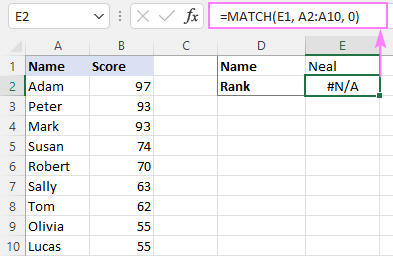Running into this error, inexperienced users might think there's something wrong with the formula, and you as the workbook creator will receive a lot of questions. To avoid this, you can explicitly indicate that the formula is correct, it just cannot find the value it is asked to look for. So, you nest the MATCH formula in the first argument of IFNA and, in the second argument, type your custom text, "Not found" in our case:

`=IFNA(MATCH(E1, A2:A10, 0), "Not found")`

Now, instead of the standard error notation, your own text is displayed in a cell, informing users that the lookup value is not present in the dataset: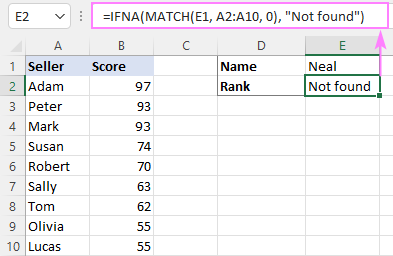## How to use IFNA with VLOOKUP

Most often the #N/A error occurs in functions that look for something such as VLOOKUP, HLOOKUP, LOOKUP, and MATCH. The below examples cover a few typical use cases.

### Example 1. Basic IFNA VLOOKUP formula

To trap #N/A errors that occur when VLOOKUP is unable to find a match, check its result using IFNA and specify the value to be displayed instead of the error. The common practice is to wrap the IFNA function around your existing VLOOKUP formula using this syntax:

In our sample table, suppose you want to retrieve a score of a particular student (E1). For this, you are using this classic VLOOKUP formula:

`=VLOOKUP(E1, A2:B10, 2, FALSE)`

The issue is that Neal did not take the exam, therefore his name is not in the list, and obviously VLOOKUP fails to find a match.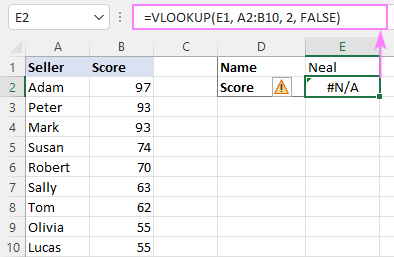To hide the error, we wrap VLOOKUP in IFNA like this:

`=IFNA(VLOOKUP(E1, A2:B10, 2, FALSE), "Did not take the exam")`

Now, the result does not look so intimidating to the user and is a lot more informative: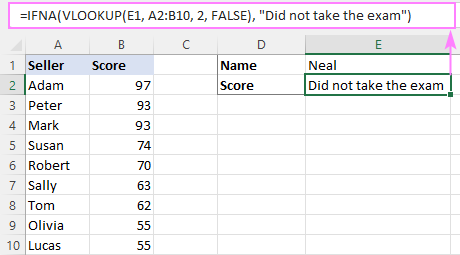### Example 2. IFNA VLOOKUP to look up across multiple sheets

The IFNA function also comes in handy for performing the so-called sequential or chained lookups across multiple sheets or different workbooks. The idea is that you nest a few different IFNA(VLOOKUP(…)) formulas one into another in this way:

If a primary VLOOKUP does not find anything, its IFNA function runs the next VLOOKUP until the desired value is found. If all lookups fail, the formula will return the specified text.

Supposing you have the scores of different classes listed in different sheets (named Class A, Class B, and Class C). Your goal is to get the score of a particular student, whose name is input in cell B1 in your current worksheet. To fulfil the task, make use of this formula:

`=IFNA(VLOOKUP(B1, 'Class A'!A2:B5, 2, FALSE), IFNA(VLOOKUP(B1, 'Class B'!A2:B5, 2, FALSE), IFNA(VLOOKUP(B1, 'Class C'!A2:B5, 2, FALSE), "Not found")))`

The formula sequentially looks up for the specified name in three different sheets in the order VLOOKUP's are nested and brings the first found match: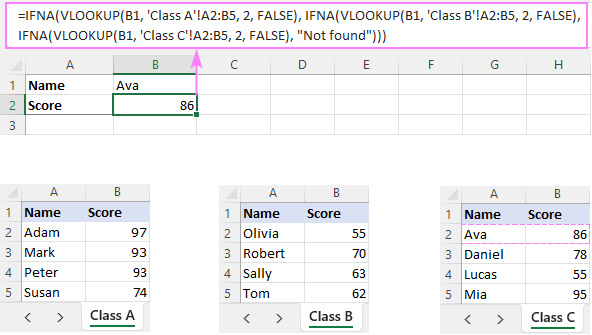### Example 3. IFNA with INDEX MATCH

In a similar fashion, IFNA can catch #N/A errors generated by other lookup functions. As an example, let's use it together with the INDEX MATCH formula:

`=IFNA(INDEX(B2:B10, MATCH(E1, A2:A10, 0)), "Not found")`

The gist of the formula is the same as in all previous examples - INDEX MATCH performs a lookup, and IFNA evaluates the result and catches a #N/A error if the referenced value is not found.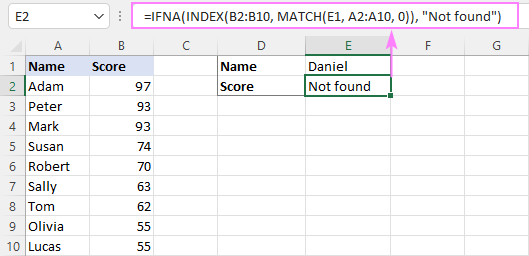## IFNA to return multiple results

In case the inner function (i.e. the formula placed in the value argument) returns multiple values, IFNA will test each returned value individually and output an array of results. For example:

`=IFNA(VLOOKUP(D2:D4, A2:B10, 2, FALSE), "Not found")`

In Dynamic Array Excel (Microsoft 365 and Excel 2021), a regular formula in the topmost cell (E2) spills all the results in the neighboring cells automatically (in terms of Excel, it's called a spill range).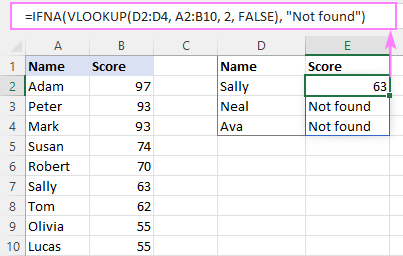In pre-dynamic versions (Excel 2019 and lower), a similar effect can be achieved by using a multi-cell array formula, which is completed with the Ctrl + Shift + Enter shortcut.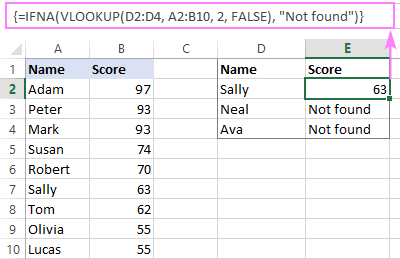## What's the difference between IFNA and IFERROR?

Depending on the root cause of the problem, an Excel formula can trigger different errors such as #N/A, #NAME, #VALUE, #REF, #DIV/0, #NUM, and others. The IFERROR function catches all those errors while IFNA is limited to only #N/A. Which one is better to choose? That depends on the situation.

If you want to suppress any kind of error, then use the IFERROR function. It is especially useful in complex calculations when a formula includes several functions that can generate different errors.

With lookup functions, you'd better use IFNA as it displays a custom result only when a lookup value is not found and does not hide underlying problems with the formula itself.

To illustrate the difference, let's bring back our basic IFNA VLOOKUP formula and "accidentally" misspell the function's name (VLOKUP instead of VLOOKUP).

`=IFNA(VLOKUP(E1, A2:B10, 2, FALSE), "Did not take the exam")`

IFNA does not suppress this error, so you can clearly see that something is wrong with one of the function names: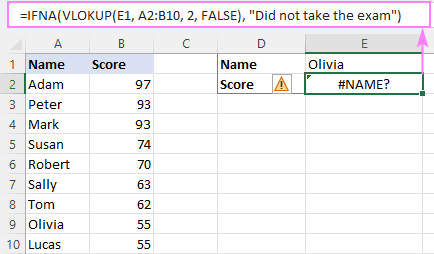Now, let's see what happens if you use IFERROR:

`=IFERROR(VLOKUP(E1, A2:B10, 2, FALSE), "Did not take the exam")`

Hmm… it says that Olivia did not take the exam, which is not true! This is because the IFERROR function traps the #NAME? error and returns the custom text instead. In this situation, it not only returns wrong information but also obscures the issue with the formula.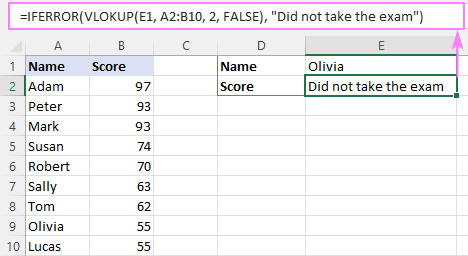That's how to use IFNA formula in Excel. I thank you for reading and look forward to seeing you on our blog next week!

Excel IFNA formula examples (.xlsx file)